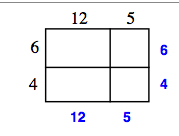### Home > CAAC > Chapter 4 > Lesson 4.2.2 > Problem4-83

4-83.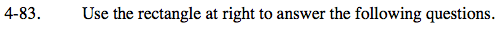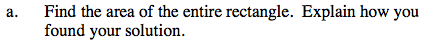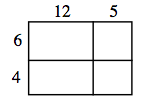Find the area of the individual parts, then sum the parts.

Find the total length of each side.

Find the area of the large rectangle by multiplying the length of each side.

170 square units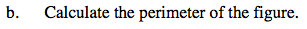Find the length of each side, then sum the sides.